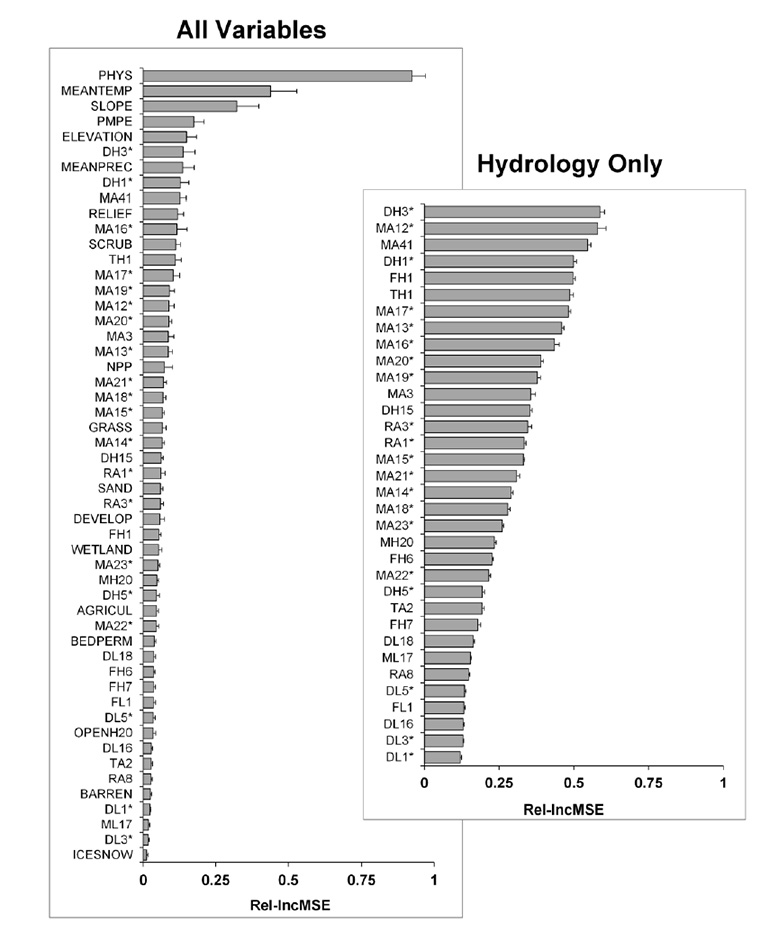Ecological Archives A025-016-A3

Ryan McManamay and Emmanuel A. Frimpong. 2015. Hydrologic filtering of fish life history strategies across the United States: implications for stream flow alteration. Ecological Applications 25:243263. http://dx.doi.org/10.1890/14-0247.1

Appendix C. A comparison of variable importance in random forest tree-models.

Variable importance for each variable was calculated from out-of-bag (OOB) observations, which were randomly permuted and then predicted using each tree. Variable importance was assessed using the proportional increase in MSE values (IncMSE), which is calculated as the average increase in MSE with the absence of each predictor in randomly permuted OOB data. IncMSE values were standardized by the maximum value for each model to compute relative values across all models (Rel-IncMSE). Rel-IncMSE values were calculated for 'all variable' models and for models using only hydrologic variables (Fig. C1).Fig. C1. Relative variable importance for "all-variable" models and "hydrology-only" models as measured by Rel-IncMSE.